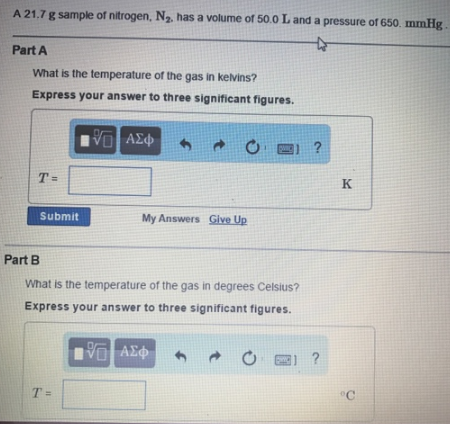# Problem: A 21.7 g sample of nitrogen. N2, has a volume of 50.0 L and a pressure of 650. mmHg.What is the temperature of the gas in Kelvins? Express your answer to three significant figures.What is the temperature of the gas In degrees Celsius? Express your answer to three significant figures.

###### FREE Expert Solution
80% (266 ratings)###### Problem Details

A 21.7 g sample of nitrogen. N2, has a volume of 50.0 L and a pressure of 650. mmHg.

What is the temperature of the gas in Kelvins?

What is the temperature of the gas In degrees Celsius?